# Bihar Board Class 12 Physics Solutions Chapter 14 Semiconductor Electronics: Materials, Devices and Simple Circuits

Bihar Board Class 12 Physics Solutions Chapter 14 Semiconductor Electronics: Materials, Devices and Simple Circuits Textbook Questions and Answers, Additional Important Questions, Notes.

## BSEB Bihar Board Class 12 Physics Solutions Chapter 14 Semiconductor Electronics: Materials, Devices and Simple Circuits

### Bihar Board Class 12 Physics Semiconductor Electronics: Materials, Devices and Simple Circuits Textbook Questions and Answers

Question 1.
In an n-type silicon, which of the following statement is true –
(a) Electrons are majority carriers and trivalent atoms are the dopants.
(b) Electrons are minority carriers and pentavalent atoms are the dopants.
(c) Holes are minority carriers and pentavalent atoms are the dopants.
(d) Holes are majority carriers and trivalent atoms are the dopants.
(c) Holes are minority carriers and pentavalent atoms are the dopants.

Question 2.
Which of the statement given in Exercise 14.1 is true for p-type semiconductors?
(b) Holes are majority carriers and trivalent atoms are the dopants.

Question 3.
Carbon, silicon and germanium have four valence electrons each. These are characterised by valence and conduction bands separated by energy band gap respectively equal to (Eg)c, (Eg )si and (Eg)Ge  Which of the following statements is true?
(a) (Eg)si. < (Eg)Ge < (Eg)C
(b) (Eg)C<(Eg)Ge< (Eg)si
(c) (Eg)C > (Eg)si > (Eg)Ge
(d) (Eg)C = (Eg)si > = (Eg)Ge
(c) (Eg)C>(Eg)si>(Eg)Ge

Question 4.
In an unbiased p-n junction, holes diffuse from the p- region to n-region because
(a) free electrons in the n-region attract them.
(b) they move across the junction by the potential difference.
(c) hole concentration in p-region is more as compared to n- region.
(d) All the above.
(c) Hole concentration in p-region is more as compared to n-region.

Question 5.
When a forward bias is applied to p-n junction, it
(a) raises the potential barrier.
(b) reduces the majority carrier current to zero.
(c) lowers the potential barrier.
(d) None of the above
(c) Lowers the potential barrier.Question 6.
For transistor action, which of the following statements are correct:
(a) Base, emitter and collector regions should have similar size and doping concentrations.
(b) The base region must be very thin and lightly doped.
(c) The emitter junction is forward biased and collector junction is reverse biased. .
(d) Both the emitter junction as well as the collector junction are forward biased.
(b) The base region must be very thin and lightly doped.
(c) The emitter junction is forward biased and collector junction is reverse biased.

Question 7.
For a transistor amplifier, the voltage gain
(a) remains constant for all frequencies.
(b) is high at high and low frequencies and constant in the middle frequency range.
(c) is low at high and low frequencies and constant at mid frequencies.
(d) None of the above
(c) Is low at high and low frequencies and constant at mid frequencies.

Question 8.
In half-wave rectification, what is the output frequency if the input frequency is 50 Hz. What is the output frequency of a full-wave rectifier for the same input frequency.
A half wave rectifier rectifies only the half of the A.C. input i.e. it conducts once during an A.C. input cycle.
∴ frequency of A.C output = frequency of A.C input = 50 Hz.
On the other hand, a full-wave rectifier rectifies both the half cycles of the A.C. input i.e. it conducts twice during a cycle.
So frequency of A.C. output = 2 x frequency of A.C. Input.
= 2 x 50 = 100 Hz.

Question 9.
For a CE-transistor amplifier, the audio signal voltage across the collected resistance of 2 KΩ is 2 V, Suppose the current amplification factor of the transistor is 100, find the input signal voltage and base current, if the base resistance is 1KΩ.
Here, (P) = current gain = 100
Ri = input (base) resistance = 1 KΩ = 1000 Ω
R0 = output (collector) resistance = 2 KΩ = 2000 Ω
V0 = output voltage = 2V
∴ Resistance again = $$\frac{\mathrm{R}_{\mathrm{o}}}{\mathrm{R}_{i}}=\frac{2000}{1000}$$ = 2
Using, the relation
Voltage gain = current gain x resitance gain,
We get, Voltage gain Av = 100 x 2 = 200
Collector current, Ic = $$\frac{\mathrm{V}_{\mathrm{o}}}{\mathrm{R}_{\mathrm{o}}}$$
= $$\frac{2 \mathrm{~V}}{2000 \Omega}=\frac{1}{1000}$$ A
= 10-3A = 1mA
Ib = base current = ?
V1 = input signal voltage = ?
Using the relation,
β = $$\frac{\mathrm{I}_{\mathrm{c}}}{\mathrm{I}_{\mathrm{b}}}$$
Ib = $$\frac{I_{c}}{\beta}=\frac{10^{-3}}{100}$$
= 10-5A = 10 x 10-6A
= 10 µA
Also using the relation, ‘
Av = $$\frac{\mathrm{V}_{\mathrm{o}}}{\mathrm{V}_{\mathrm{i}}}$$
= $$\frac{V_{o}}{A_{v}}=\frac{2}{200}=\frac{1}{100}$$ V
= 0.01 V

Question 10.
Two amplifiers are connected one after the other in series (cascaded). The first amplifier has a voltage gain of 10 and the second has a voltage gain of 20. If the input signal is 0.01 volt, calculate the output ac signal.
Let Av1 and Av2 be the voltage gains of the first and second amplifier connected in series.
∴ Av1 = 10
Av2 = 20
Vi = input, A.C signal voltage = 0.01 V.
Vc = output, A.C signal voltage = ?
Let Av be the voltage gain of the cascaded amplifier.
∴ A v = Av1 x Av2
= 10 x 20 = 200

∴ using the relation,
Av = $$\frac{\mathrm{V}_{\mathrm{o}}}{\mathrm{V}_{\mathrm{i}}}$$ , we get,
V0 = Av x Vi = 200 x 0.01
= 2 VQuestion 11.
A p-n photodiode is fabricated from a semiconductor with band gap of 2.8 eV. Can it detect a wavelength of 6000 nm?
Here
Eg = band gap of the semiconductor
= 2.8 eV
= 2.8 x 1.6 x 10-19 J
= 4.48 x 10-19 J
= 44.8 x 10-20 J ……… (1)
λ = 6000 nm = 6000 x 10-19 m
If E be the energy corresponding to this radiation, then
E = $$\frac{\mathrm{hc}}{\lambda}$$
= $$\frac{\mathrm{hc}}{\lambda}$$
= 3.31 x 10-20J ……..(2)
It is clear from (1) and (2), that the energy corresponding to 6000 nm is less than the band of the semiconductor used to fabricate photodiode, so it cannot be detected. For detection of any radiation, E > Eg.

Question 12.
The number of silicon atoms per m3 is 5 x 1028. This is doped simultaneously with 5 x 1022 atoms per m3 of Arsenic and 5 x 102 per m3 atoms of Indium. Calculate the number of electrons and holes. Given that n x = 1.5 x 1016 m-3. Is the material n – type or p – type?
Here,
ND = 5 x 1020 atoms/m3
= donor atom density
NA= Acceptor atom density = 5 x 1020 atoms/m3
NA = 0.05 x 1022 atoms/m3
ni = 1.5 x 1016m-3
N = number of silicon atoms = 5 x 1028 m-3
Let ne and nh be the number density of electrons and holes respectively in the semiconductor = ?
∴ ND – NA = (5 – 0.05) x 1022
= 4.95 x 1022 atoms/m3
We know that
nenh = n2i ……… (1)
Also know that for the neutrality of charge in the semiconductor,
ND – NA = ne – nh
or ne – nh = ND – NA ……….(2)
From(1)
nh = $$\frac{\mathrm{n}_{\mathrm{i}}^{2}}{\mathrm{n}_{\mathrm{e}}}$$ ……….(3)
∴ From (2) and (3), we get
$$\frac{\mathrm{n}_{\mathrm{i}}^{2}}{\mathrm{n}_{\mathrm{e}}}$$ = ND – NA
or (ND – NA) ne = $$\frac{\mathrm{n}_{\mathrm{i}}^{2}}{\mathrm{n}_{\mathrm{e}}}$$ ……..(4)
eq (4) is quadratic equation in ne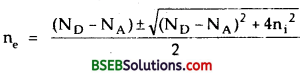neglecting negative sign before square root because otherwise ne becomes zero, we get[∴ n1 is very small, so n12 will be very small as compared to (ND – NA)2, hence can be neglected].
∴ ne = – [4.95 x 1022 + 4.95 x 1022]
= 4.95 x 1022 m-3
from (3),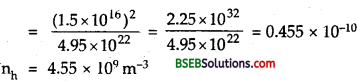Clearly as ne >> nh, i.e. electrons are majority carriers and holes are minority carriers in the given semiconductor, so it is of n-type.

Question 13.
In an intrinsic semiconductor the energy gap Eg is 1.2 eV. Its hole mobility is much smaller than electron mobility and independent of temperature. What is the ratio between conductivity at 600K and that at 300K? Assume that the temperature dependence of intrinsic carrier concentration n1 is given by
ni = n0 exp – $\frac{\mathbf{E}_{\mathbf{g}}}{\mathbf{2 K}_{\mathbf{B}} \mathbf{T}}$
where n0 is a constant.
Here, Eg= energy gap of the intrinsic semiconductor = 1.2 eV
T1 = 600K ‘
T2 = 300K
Let σ1 and σ2 be the conductivity of the semiconductor at T1, and T2repectively = ?
∴ $$\frac{\sigma_{1}}{\sigma_{2}}$$ = ?
We know that the conductivity of a semiconductor is given by –
σ = $$\frac{1}{ρ}$$
e = e(neµe + nnµn) ……..(1)
For intrinsic semiconductor, ne = nh = ni
also µe>> un (given)
∴ eg (1) can be written as :
σ = e ni ne ……….(2)
Also it is given that
ni = n0 e$$e^{-E g / 2 k B T}$$ ………(3)
From (2) and (3), we get
where σ = e n0u0 $$e^{-E g / 2 k B T}$$
= σ0 $$e^{-E g / 2 k B T}$$ ………(4)
where σ0 = e n0u0 is a constant as it is independent of temperature.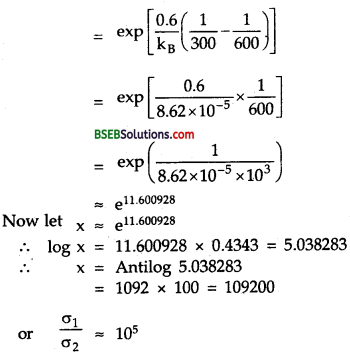From it, we conclude that the conductivity of the intrinsic semiconductor is highly affected by temperature.

Question 14.
In a p-n junction diode, the current I can be expressed as
I = I0 exp ($$\frac{\mathrm{eV}}{\mathrm{K}_{\mathrm{B}} \mathrm{T}}-\mathbf{1}$$)
where I0 is called the reverse saturation current, V is the voltage across the diode and is positive for forward bias and negative for reverse bias, and I is the current through the diode, KB is the Boltzmann constant (8.6 x 10-5 eV/K) and T is the absolute temperature. If ior a given diode I0 = 5x 10-12 A and T = 300 K, then
(a) What will be the forward current at a forward voltage of 0.6 V?
(b) What will be the increase in the current if the voltage across the diode is increased to 0.7 V?
(c) What is the dynamic resistance?
(d) What will be the current if reverse bias voltage Changes from 1 V to 2 V?
Here, kB -Boltzmann constant = 8.6 x 10-5 eVK-1
= 8.6 x 10-5 x 1.6 x 10-19jK-1
I0 = 5 x 10-12,
A = reverse saturation current.
T = 300 K.

(a) Here, V = 0.6V
forward current is given byNow at
X = (2.718)23.255
∴ log x = 23.255 log (2.718)
= 23.255 x 0.4343 = 10.09965
∴ x = Antilog 10.0997 = 10.0997
= 1258 x 107 ………(2)
∴ From (1) and (2), we get
I = 5×1012 x (1258 x 107 – 1)
= 6290 x 105 A (neglecting one)
= 0.0629A = 0.063 A

(b) Here, V = 0.7V
Let I be the forward current when forward voltage is increased to 0.7V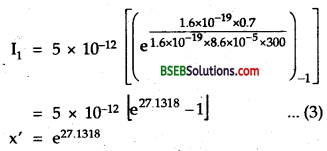log x’ = 27.1318 log e
= 27.1318 x 0.4343
= 11.7833
x’ = Antilog 11.7833
= 6072 x 108 ………..(4)
from (3) and (4), we get
I1 = 5 x 10-12 x (6072 x 108 – 1)
= 5 x 10-12 x 6072 x 108
= 30360 x 108
= 3.036 A
If ∆I be the increase in current, then
∆I = I1 – I = 3.036 – 0.063 = 2.973 A.

(c) $$\frac{\Delta \mathrm{V}}{\Delta \mathrm{I}}$$ = dynamic resistance = Rd = ?
Here
∆V = 0.7 – 0.6 = 0.1V
∆I = 2.973 A
∴ Rd = $$\frac{\Delta \mathrm{V}}{\Delta \mathrm{I}}$$
= $$\frac{0.1}{2.973}$$
= 33.636 x 10-3
= 0.0336 Ω

(d) For a change in voltage from 1 to 2V, the current will remain to saturation state,
I0 = 5 x 10-12A.
It shows that the diode possesses practically infinite resistance in reverse biasing.Question 15.
You are given the two circuits as shown in Fig here. Show that circuit (a) acts as OR gate while the circuit (b) acts as AND gateIn fig (a), y1 = $$\overline{\mathrm{A}+\mathrm{B}}$$
and y = $$\overline{y_{1}}=\overline{\overline{A+B}}$$ $$\overline{\overline{\mathrm{A}}}+\overline{\overline{\mathrm{B}}}$$ = A + B
i.e. Y equal to A OR B
Hence fig (a) represents an OR gate and is verified by the following truth tablei.e y, is high if both the inputs are low and low if one or both the inputs are high i.e; y1 is the output of a NOR gate.
For an OR gate the output is high if one or both the inputs are high and low if one or both the inputs are low.
Now from table, we see that Y is O i.e. low when both A and B are low and is high when either A or B or both are high. Hence it is the truth table of OR gate.
(b) In fig. (b), y1 = $$\overline{\mathrm{A}}$$
y2 = $$\overline{\mathrm{B}}$$
and y = $$\overline{y_{1}+y_{2}}$$
= $$\overline{y_{1}} \cdot \overline{y_{2}}$$
[Using De-Morgan’s theorem $$\overline{\mathrm{A}+\mathrm{B}}$$ = $$\overline{\mathrm{A}} \cdot \overline{\mathrm{B}}$$ ]
= $$\overline{\overline{\mathrm{A}}}+\overline{\overline{\mathrm{B}}}$$ = A.B
i.e.; y equals A AND B.
Clearly Fig (b) represents an AND gate and is also verified by the following truth table.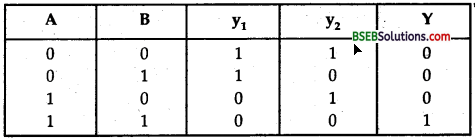Hence, y is the output of the NOR gate having y1 and y2 as inputs. For NOR gate the output is high if both the inputs are low and the output is low if one or both the inputs are high. Thus clearly Y represents the truth table of an AND gate for which the output is high if both the inputs are high and the output is low if one or both the inputs are low.

Question 16.
Write the truth table for a NAND gate connected as given in following figure.Hence identify the exact logic operation carried out by this circuit
The NAND gate shown here has only one input and one output i.e. the two inputs are shorted. For a NAND gate, the output is high if one or both the inputs are low and the output is low if both the inputs are high. Thus its truth table is given as :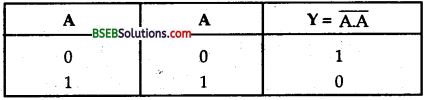It is clear that here the logic operation
Y = $$\overline{\mathrm{A}}$$ is taking place.
So this circuit carries out the operation of a NOT gate actually having the truth table.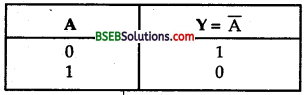Question 17.
You are given two circuits as shown in Fig. here which consist of NAND gates. Identify the logic operation carred out by the two circuits.
(a) fig a, A and B are the inputs to NAND gate having y: as its output. yx is input to NAND gate acting as NOT gate i.e.
y = $$\overline{y_{1} \cdot y_{1}}$$ = $$\overline{y_{1}}$$
y1 = $$\overline{\text { A.B }}$$
∴ y = $$\overline{\overline{\mathbf{A} . \mathbf{B}}}$$ = A. B
which is an AND operation and is explained in theJFollowing truth table.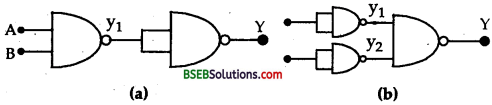(b) In figure (b) A and B are the inputs to the two separate NOT gates obtained from NAND gates and have their respective outputs as y1 and y2 which are inputs to another NAND gate having output y.
Thus y = $$\overline{y_{1} \cdot y_{2}}$$
Also y1 = $$\overline{\text { A.A }}$$ = $$\overline{\mathbf{A}}$$
and y2 = $$\overline{\mathrm{B}}$$.$$\overline{\mathrm{B}}$$ = $$\overline{\mathrm{B}}$$
y = $$\overline{\overline{\mathrm{A}}}$$ . $$\overline{\overline{\mathrm{B}}}$$ = $$\overline{\overline{\mathrm{A}}}$$ + $$\overline{\overline{\mathrm{B}}}$$ (by using De-Morgan’s theorem) = A + B
which is an OR operation.
Thus this figure represents an OR gate.
The operations carried out by this circuit are shown in the , following truth table.For OR gate the output is high if one or both the inputs are high and the output is low if both the inputs are low.
Thus the table is correct with A and B as inputs and y as output.

Question 18.
Write the truth table for circuit given in Fig. below consisting of NOR gates and identify the logic operation (OR, AND, NOT) which this circuit is performing.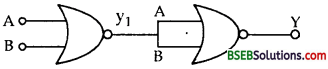(Hint – A = 0, B = 1, then A and B inputs of second NOR gate will be O and hence y = 1. Similarly work out the values of y for other combinations of A and B. Compare with truth of OR, AND, NOT gates and find the correct one.
Here, the above figure represents a NOR gate followd by a NOT gate obtained from NOR gate. Thus the output y, will represent the output of a NOR gate and is fed to NOT gate. Thus we get an OR gate as shown in the truth table and the Boolean operations carried out are also given here.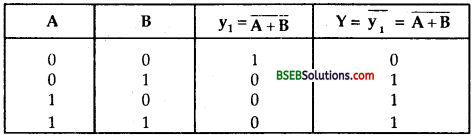y = $$\overline{y_{1}}$$ = $$\overline{\overline{A+B}}$$ = A + B (∵ $$\overline{\overline{\mathrm{A}}}$$ = A)
Thus A, B and Y represents the table of OR gate so it is the circuit-‘ requivalent to OR gate i.e.; when A = B = 0, then yx will be high because for a NOR gate the output is high if both the inputs are low and the output is low if one or both the inputs are high.
Similarly when A = 0, B = 1, y1 = 0
A = 1, B = 0, y1 = 0
and A = B = 1, y1 = 0.

Question 19.
Write the truth table for the circuits given in figure consisting of NOR gates only. Identify the logic operations (OR, AND, NOT) performed by the two circuits.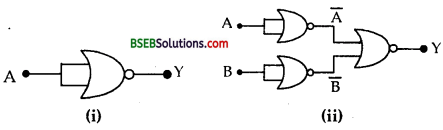(a) Here, fig (a) represents a NOR gate having its two inputs shorted followed by a NOT gate. The Boolean expression and Truth Table are given below.
y = $$\overline{\mathrm{A}}$$
For a NOR gate, the output is low if one or both the inputs are high and the output is high if both the inputs are low.
Thus clearly this circuit carries out the logic operation of a NOT gate.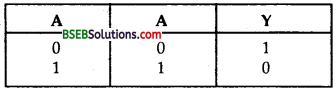Or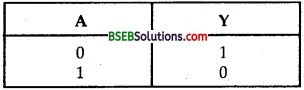(b) This circuit shows how NOR gate acts as an AND gate. Here for 1st two gates the input terminals are joined together and act as NOT gates which invert the inputs A and B. Their output is now jointly fed to the NOR gate. The output so obtained is that of the AND gate. The truth table for the circuit and the Boolean expressions are given below.Thus with inputs as A and B and output as y: we get the truth table of AND gate
y = $$\overline{\overline{A+B}}$$ = $$\overline{\overline{\text { A }}} \cdot \overline{\overline{\mathrm{B}}}$$ = A. B

### Bihar Board Class 12 Physics Semiconductor Electronics: Materials, Devices and Simple Circuits Additional Important Questions and Answers

Question 1.
Name the charge carriers in the following at room temperature.

1. Conductors
2. Intrinsic semiconductors
3. Insulators

1. In conductors the charge carriers are electrons.
2. In intrinsic semiconductors the charge carriers are electrons and holes.
3. In insulators there are no charge carriers.

Question 2.
Out of Ge and Si which is better semiconductor and why?
Ge is better semiconductor because Eg for it (0.8 eV) is smaller than Eg for Si (1.1 eV). So conduction in Ge can take place more easily.

Question 3.
Draw energy band diagram for n-type extrinsic semiconductor.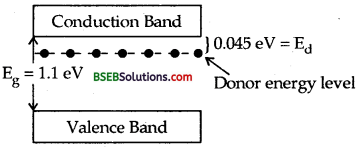Question 4.
What type of semiconductor do you obtain by doping
(i) Si with Indium
(ii) Ge with Sb?
(i) Doping Si with Indium means formation of extrinsic semiconductor in which trivalent impurity has been added which results in p-type semiconductor.
(ii) Doping Ge with Sb (antimony) means formation of extrinsic semiconductor in which pentavalent impurity has been added which results in n-type semiconductor.

Question 5.
Give symbol of (i) p-n junction (ii) p-n-p transistor, (iii) n- p-n transistor.
(i) It is shown below p-region. is known as anode and n- region is known as cathode.Symbol of p-n junction or semiconductor diode.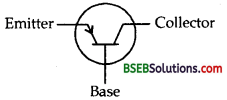The arrow points in the direction of conventional current i.e. in the direction of flow of hole current.

(ii) Symbol of p-n-p transistor.(iii) Symbol of n-p-n transistor.

Question 6.
Draw the circuit diagram of (i) half wave rectifier, (ii) full- wave rectifier.
The circuit diagram of half wave and full wave rectifiers are shown in Fig. (a) and (b) respectively.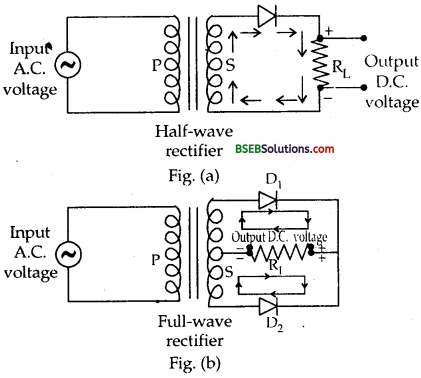Question 7.
Give three differences between intrinsic and extrinsic semiconductors.
Intrinsic Semiconductor :

• Available in pure form.
• Conductivity is low
• Number of electrons and holes are equal.
∴ ne = na

Extrinsic Semiconductor :

• Doped with suitìb1e impurities
• Conductivitity is high
• There is either mority of electrons (n-type) or napority of hole (p-type)
∴ ne ≠ nh

Question 8.
Output of AND gate is connected to input of NOT gate. Draw the truth table of combination and what is it called
The truth table of the combination is given below:If is called NANDgate

Question 9.
Give symbol, Boolean equation and truth table of OR gate.
Symbol of OR ga te is.Boolean equation is : Y = A + B read as Y equals A or B. The Truth Table isQuestion 10.
What are α and β ? Define them.
α is the current gain of the common base configuration and p is the current gain of the common emitter configuration.
α : It is defined as the ratio of the change in collector current to the
change in emitter current i.e. α =$$\frac{\Delta I_{c}}{\Lambda I_{e}}$$
β : It is defined as the ratio of the change in collector current to the change in base current, i.e, β = $$\frac{\Lambda I_{\mathrm{c}}}{\Lambda \mathrm{I}_{\mathrm{b}}}$$Question 11.
Why is semiconductor damaged when strong current is passed ?
A strong current when passed through a semiconductor heats it up and covalent bonds break. This breakage of bonds results in large number of free electrons and the semiconductor behaves as a conductor. As the semiconductor has lost the property of low conduction, it is said to be damaged.

Question 12.
Explain why a transistor is a current operated device?
The action of a transistor is controlled by the charge carriers i.e.; electrons and holes, hence it is a current operated device.

Question 13.
In the following circuits, which of the diode is forward biased and which is reverse biased?n of p-n j unction is at +5 V and p of junction is at 10 V or p is at + 5 V w.r.t. n. The diagram looks like clearly as D1 is foward biased.n is at – 12 V and p Ls -5V or p is at -5V- (-12V) = 7 V w.r.t. n. Clearly D2 is also forward biased.

Question 14.
Why are the NAND or NOR gates called as a digital building blocks?
They are called so because the repeated use of NAND (or NOR) gates can produce all the three basic gates i.e. AND, OR and NOT gates that are used to provide large number of complicated circuits.

Question 15.
What are advantages of integrated circuits over conventional electronic circuits.

• ICs are highly reliable.
• These require small power.
• They have lower total cost.

Question 16.
What is Zener Diode? Give its symbol and one most important property.
A Zener Diode is a specially designed junction diode which can operate continuously without getting damaged when operating in reverse bias region.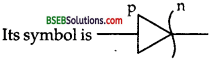For input voltage greater than Zener voltage, this diode acts as excellent voltage regulator and provides constant output voltage for any input voltage.

Question 17.
Define doping.
It is defined as the process of deliberately adding impurity atoms in an intrinsic semi-conductor to alter its properties.

Question 18. :
What is the effect of doping on the activity of semiconductor?
Doping of a semiconductor increases the electrical conductivity of the semiconductor to a large extent by creating charge carriers.

Question 19.
What are the requirements of doping process ?
(i) The size of dopant atoms must be the same as that of the crystal atoms.
(ii) The presence of dopant atoms should not distort the crystal lattice.
(iii) The concentration of dopant atoms should not be high.Question 20.
Define temperature coefficient of resistance of a semiconductor.
It is defined as the change in resistance of a semiconductor with the temperature. They have negative temperature coefficient of resistance as their resistance decreases with the increase in temperature.

Question 21.
Define a p-n function diode or semiconductor diode.
It is defined as the combination obtained by placing p-type semiconductor in contact with an n-type semiconductor.

Question 22.
Define depletion region.
It is defined as the thin layer or region at the junction which is devoid of (depleted of) mobile charge carriers (i.e. electrons and holes.)

Question 23.
Define potential barrier.
It is defined as the potential difference developed across the p-n junction due to the migration of majority charge carriers.

Question 24.
What is the function of potential barrier in a p-n junction?
The function of the potential barrier in a p-n junction is to stop the further migration of majority charge carriers from one region of p-n junction to another. It repels the incoming charge carriers.

Question 25.
What is the effect of forward bias voltage on
(a) the thickness of depletion layer.
(b) resistance of the junction?
(a) The thickness of the depletion layer decreases due to forward biasing.
(b) The resistance of the function also decreases due to forward biasing.

Question 26.
(a) Is the value of potential barrier for a given p-n junction diode fixed?
(b) On what factors does it depend?
(a) No, its value is not fixed.
(b) The value of potential barrier depends upon the following factors:
1. Nature of the semiconductor cyrstal.
2. Temperature of the crystal.
3. Extent of doping of the semicond uctor crystal.Question 27.
Define Zener voltage. On what factors does it depend?
It is defined as the maximum reverse voltage which a diode can withstand without its breakdown. It depends on the density of impurity atoms.

Question 28.
Define (a) rectifier, (b) rectification of a.c. power.
(a) It is defined as a device which converts a.c. power into d ,c. power
(b) It is defined as the process of converting a.c. power into d.c. power

Question 29.
Define a solar cell.
It is defined as a p-n junction diode which converts sun light directly to electrical energy. It has large conversion efficiency.

Question 30.
Explain the function of each part in a transistor.
Emitter supplies the majority carriers for the flow of current. Collector collects the majority carriers emitted by the emitter. The base provides the junction for interaction between the emitter and the collector.

Question 31.
What are the uses of solar cells?

• They are used in satellites to provide electrical ener gy.
• They are used in calculators, wrist watches etc. as a source of power.
• They are used for charging storage batteries in day time which can be used during the night to supply power.

Question 32.
(a)Define knee voltage.
(b)ripple factor of rectifier.
(a) It is defined as the forward bias beyond which the current through the junction starts increasing rapidly with the applied voltage. It is also called cut-in voltage.
(b) It is defined as tire ratio of the value of a.c. component to the value of d.c. component.

Question 33.
Define
(a) input characterstics
(b) Output characteristics of a transistor.
(a) They are defined as the graphs between the input voltage and input current at a given value of the collector voltage.
(b) They are defined as the graphs between collector voltage and collector current at constant values of base current i.e.; input current.

Question 34.
Differentiate between Wafer and chip.
Wafer: It is defined as a thin slice of semiconductor crystal of about 0.05 cm thickness and diameter ranging from 2.5 cm to 10 cm
Chip : It is defined as a small part of semiconductor wafer of size 12.7 x 12.7 mm2

Question 35.
What are the advatages of integrated circuits.

• They a re of lost cost.
• They are highly reliable.
• They require very small space.

Question 36.
Why transistor is preferred to be used as an amplifier in common emitter configuration?
It is so because the current gain is very high in common emitter amplifier as compared to common base amplifier.

Question 37.
Define dynamic resistance or a.c. resistance of a junction diode.
It is defined as the ratio of small change in voltage to the small change in current.
i.e. rb = $$\frac{\Delta \mathrm{V}}{\Delta \mathrm{I}}$$

Question 38.
Define transconductance.
It is defined as the ratio of change in collector (output) current . to the change in the base emitter (input) voltage.
i.e. Mathematically
δm = $$\frac{\Delta \mathrm{I}_{\mathrm{c}}}{\Delta \mathrm{V}_{\mathrm{be}}}$$Question 39.
Why the base of a transistor is always made thin and lightly doped?
The base of a transistor is always made thin and lightly doped so as to minimise the neutralisation (= 5%). When the charge carriers cross the base
region, they combine with charge carriers of opposite nature and get neutralised.

Question 40.
Define a binary number system.
It is a simple alternative way to count and express numbers. It makes use of only two digits i.e.; 0 and 1 to represent any number.

Question 41.
Define a logic gate.
It is defined as a digital circuit with one or more than one input but only one output and works according to some logical relationship between the input and output voltages.

Question 42.
Define a truth table of a logic gate
It is defined as a table that shows all the input and output possibilities for the logic gate.

Question 43.
Name the three basic logic gates.
The three basic logic gates are:

• AND gate
• OR gate
• NOT gate

Question 44.
Define nibble.
A binary digit is known as bit and a group of four bits is called a nibble.

Question 45.
What is the principle of junction diode to act as a rectifier?
It works on the fact that the junction diode offers low resistance to the current when it is forward biased and does not conduct when it is reverse biased.

Question 1.
Write the truth table for the following combinations of gates.The gates marked X and X’ are OR and AND gates respectively. Let Y’ be the output of OR gate.
The truth table is as follows :Question 2.
If A is ‘off’ and B is ‘on’, show that De Morgan’s theorem is satisfied.
According to De-Morgan’s theorem,Hence De-Morgan’s theorem i is satisfied.

Question 3.
Why a NOT gate is known as an inverter circuit?
A NOT gate gives output for no input and no output for input. In other words, it puts the input conditions in the opposite order i.e., it inverts the input order. Due to this reason, a NOT gate is known as inverter circuit.

Question 4.
Convert 49 number into binary system.∴ (Decimal number)10 = (M.S.B ………. L.S.B)2
∴ (49)10 = (110001)2

Question 5.
What is a saturation state in a NOT gate?
To get the maximum collector current, the values of collector circuit resistance and the base circuit resistance are adjusted in a NOT gate. This state of the NOT gate is known as saturation state.

Question 6.
Explain why a transistor cannot be used as a rectifier?
If a transistor is to be used as a rectifier, then either emitter- base or base-collector junction has to be used as a diode. For transistor to operate, the number density of charge carriers in emitter and base or base and collector must be nearly same. As the base is thin and lightly doped, so transistor cannot work as a rectifier.Question 7.
What are the advantages of semiconductor devices over vacuum tubes.

• The semiconductor devices are shock proof.
• Their cost of production is very small.
• They can be easily transported from one place to another.
• They don’t produce humming noise.
• They have longer life.
• They are of very small size.

Question 8.
What are the differences between a p- and n-type semiconductor?
p-type semiconductor :
(i) They are obtained by adding trivalent impurity atoms in the intrinsic semiconductor.
(ii) The holes are the majority carriers.

n-type semiconductor :
(i) They are obtained by adding pentavalent impurity atoms in the intrinsic semiconductor.
(ii) The electrons are the majority carriers.

Question 9.
Derive the expression for the total current in a semiconductor?
Let l, A be the length and area of cross section of the semiconductor PQ.
V = Potential diff. applied across it.
Let I be the conventional current flowing through it. It is constituted by the flow of holes and electrons respectively.
ie., I = Ih + Ie …….(1)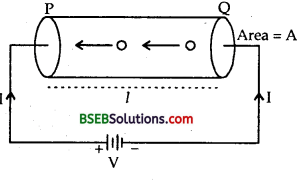Let ne nh be the electron and hole density in tfce semiconductor.
υhυe = drift speeds of holes and electrons respectively.
If q be the charge in the semiconductor due to holes from P to Q in a time t, then
q = nh x Volume of semiconductor x Charge on a hole = nh x Al x e
∴ Ih = $$\frac{q}{t}$$ = nh eA $$\frac{l}{t}$$ = nheAvn ………(2)
Similarly Ie = nh eA ve …….(3)
∴ From (1), (2) and (3), we get
I = e(nhυh + neve)A.Question 10.
Derive the expression for the electrical conductivity of the semiconductor.
Let R, p be the resistance and resistivity of the semiconductor of dimensions l and A.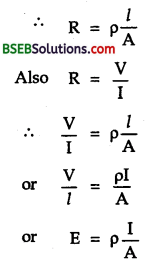When E = $$\frac{V}{l}$$ = electric field across the semiconductor due to the pot. diff. V.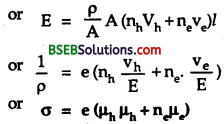which is the required expression for conductivity (σ) of the semiconductor.
where µh = $$\frac{\mathrm{v}_{\mathrm{h}}}{\mathrm{E}}$$
and µe = $$\frac{\mathbf{v}_{\mathrm{e}}}{\mathrm{E}}$$ are the mobiles of holes and electrons respectively.

Question 11.
Differentiate between the forward and reverse bias.
Forward bias :

• The junction diode is said to be forward biased when the p-and n-regions of the semiconductor are connected to positive and negative terminals of the battery.
• The thickness of the depletion layer is smaller.
• The junction diode offers low resistance to the flow of current.
• The current flow is due to the majority carriers.
• The current is measured in mA.

Reverse bias :

• The junction diode is said to be reverse biased when p-and n-regions of the semiconductor are connected to – ve and + ve terminals of the battery.
• The depletion layer width is larger.
• It offers high resistance to the flow of current.
• The current flow is due to the minority carriers.
• The current is measured in pA.

Question 12.
Define a gain factors.of C. B. amplifier.
(i) D.C. Current gain (a) – It is defined as the ratio of collector current to the emitter current i.e.,
∝ = $$\frac{\mathrm{I}_{\mathrm{c}}}{\mathrm{I}_{\mathrm{e}}}$$

(ii) A.C. Current gain – It is defined as the ratio of change in collector current to the change in emitter current at a constant collector voltage.
i.e ∝a.c = $$\left(\frac{\Delta \mathrm{I}_{\mathrm{C}}}{\Delta \mathrm{I}_{\mathrm{e}}}\right)_{\mathrm{V}_{\mathrm{Ce}}}$$ = constant

(iii) A.C Voltage gain – It is defined as the ratio of change in output voltage to the change in input voltage.
i.e Av = $$\frac{\Delta \mathbf{V}_{0}}{\Delta \mathrm{V}_{\mathrm{i}}}=\frac{\Delta \mathrm{I}_{\mathrm{c}} \cdot \mathrm{R}_{0}}{\Delta \mathrm{I}_{\mathrm{e}} \mathrm{R}_{\mathrm{in}}}$$
= ∝a.c x resistance gain
(iv) Resistance gain – It is defined as the ratio of output resistance to the input resistance.(v) A.C. power gain – It is defined as the ratio of change in output power to the change in input power.
Power gain = $$\frac{\Delta \mathrm{I}_{\mathrm{C}} \times \Delta \mathrm{V}_{0}}{\Delta \mathrm{I}_{\mathrm{e}} \times \Delta \mathrm{V}_{\mathrm{i}}}$$
= $$\frac{\left(\Delta \mathrm{I}_{\mathrm{c}}\right)^{2}}{\left(\Delta \mathrm{I}_{\mathrm{e}}\right)^{2}} \times \frac{\mathrm{R}_{0}}{\mathrm{R}_{\mathrm{in}}}$$
= ∝2a.c x resistance gain.

Question 13.
Derive the relation between a and p.
We know that
α = $$\frac{\mathrm{I}_{\mathrm{c}}}{\mathrm{I}_{\mathrm{e}}}$$
and β = $$\frac{\mathrm{I}_{\mathrm{c}}}{\mathrm{I}_{\mathrm{b}}}$$
Also we know that
Ie = Ib + Ic
Dividing on both sides by Ic we get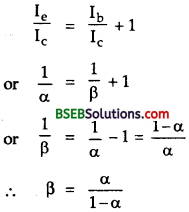Question 14.
Draw the logic symbol, truth table and the Boolean expression of the AND gate.
The logic symbol of AND gate is :truth table isThe Boolean expression is :
Y = A. B read as Y equals A AND B.

Question 15.
Draw the logic symbol, Truth table and Boolean expression for OR gate.
The logic symbol, Truth table and Boolean expression for OR gate are given below :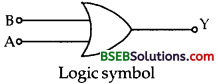truth table is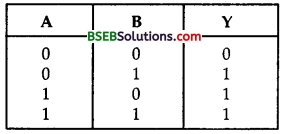Boolean expression is
Y = A + B i.e., Y equals A or B.

Question 16.
Give the logic symbol, Truth table and Boolean expression of NOT gate.
The logic symbol of NOT gate is given here :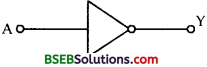It is a single input and single output gate.
The truth table of NOT gate is given below :Boolean expression is : Y = $$\overline{\mathrm{A}}$$ read as Y equals A bar.

Question 17.
All India Radio broadcastes at 710 KHz whereas BBC broadcasts at 1380 KHz on same band. For changing over from All India Radio to BBC, what change we have to make in capacitor and why ?
Since the band is same, inductor L used in tuning circuit has same value of both for All India Radio and BBC. In going from All India Radio to BBC, frequency thcreases and frequency υ = $$\frac{1}{2 \pi \sqrt{\mathrm{LC}}}$$ depends on C, υ ∝ $$\frac{1}{\sqrt{C}}$$ for same L, υ2 ∝ $$\frac {1}{C}$$ or C ∝ $$\frac{1}{v^{2}}$$ with increase in υ, clearly C has to decrease. We will have to choose capacitor of smaller capacity.

Question 18.
Differentiate between a n-p-n and p-n-p transistor.
n-p-n transistor :
(j) A thin layer of p-type semi conductor is sandwitched between two thick layers of n-type semiconductor.
(ii) Its symbol is(iii) Here emitter and collector are made up on n-type semiconductor and base is p- type.

p-n-p transistor
(i) A thin layer of n-type semi conductor is sandwitched between two thick layers of p-type semiconductor.
(ii) Its symbol is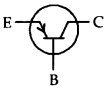(ill) Here emitter and collector are made up of p-type semiconductor and base is n type.Question 19.
The circuit shown in figure below contains two diodes each with forward resistance of 50Ω and infinite backward resistance. If the battery voltage is 6V, find the current through a 100Ω resistance.D2 is reverse biased and has infinite resistance current in arm containing D2 is zero. D1is forward biased and it conducts and offers resistance 50 Ω.Total resistance of circuit = 50 + 150 + 100 = 300 Ω
Potential difference V = 6V
Since it is series arrangement, current is same everywhere.
.’. Current through 100 Ω resistance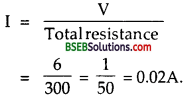Question 20.
Explain exclusive OR (i.e., XOR) gate.
An XOR gate is obtained by using AND, OR and NOT gates as shown below along with its logic symbol.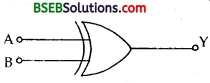Its Truth table is given below :It is dear from this table that the output is high only when the two inputs are different and the output is low if two inputs are either low or high i.c., if they are similar.

Question 21.
Explain the action of a transistor.
Consider p-n-p transistor with proper biasing of its two functions as shown below :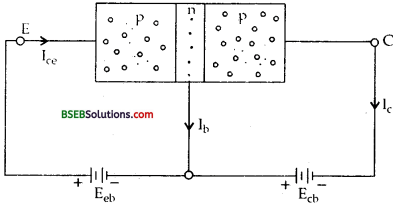Holes are majority carriers in p-type emitter and electrons are minority carriers. The holes are repelled by the Eeb towards the base region due to forward bias. The base is thin and lightly doped. When these holes enter into the base region, then nearly 5 % of them combine with electrons and the remaining i.e.,( ≈ 95 %) of holes reach the collector region due to the reverse bias of the c-b junction.

As one hole reaches the collector, an electron leaves the – ve pole of the c-b battery VCb) and combines with it, at the same time, an electron is released from some covalent bond in the emitter creating a hole in the emitter. The electron so released enters the + ve pole of the e-b battery. Thus we see that the current in p-n-p transistor is carried by holes inside the semiconductor as well as in the external circuit. Clearly
Ie = Ib + IcQuestion 1.
Explain C-B Amplifier.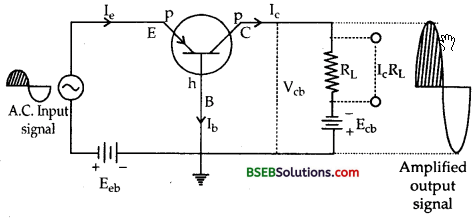The circuit diagram of C.B. pnp transistor amplifier is shown in the figure above.
In the absence of a.c. input signal, the various currents and the bias voltages are related as:
Ie = Ib + Ic ……..(1)
and Vcb, = Ecb – IcRL ……..(2)
Where Ecb = fixed collector bias and Vcb is the collector voltage i.e. voltage across the collector and emitter.
Let us now apply the a.c. input voltage across eb junction.
During its + ve half input cycle, the forward bias at the e-b junction
increases, thus Ie Ic and hence lc RL increase, so Vcb will decrease according to equation (2).
Now as the collector is already at the – ve potential, so a decrease in Vcb means that the – ve potential at the collector decreases which can be possible if we get + ve half output cycle. Similarly during – ve half input cycle, the forward bias of e.b. junction decreases which causes a decrease in Ie, Ic and hence in IcRL leading to an increase in Vcb.

Now as C is already at – ve potential, so an increase in Vcb means that the – ve potential at collector increases which can be possible if we get – ve half of output voltages. Thus we conclude that the input and output signals are in the same phase in a common base amplifier.

Question 2.
Explain common emitter (CE) amplifier.
The circuit diagram of a CE n-p-n transistor amplifier is shown in the figure here. In the absence of A.C. input signal, the various currents and bias voltages are related as :
Ie = Ib + Ic …….(1)
and Vce= Ece – IcRL …….(2)
Where Vce = collector voltage w.r.t. emitter.Let us now apply the A.C. input signal to be amplified.
During +ve half of A.C. input cycle, the forward bias of e-b junction increases leading to an incr ease in Ie, Ic and Ic RL and a decrease in Vce according to equation (a). Now as the collector is already at ; ve potential, so a decrease in Vce means that the positive potential at C decreases which can be possible if we get -ve half output A.C. cycle.
Similary during – ve half input A.C. cycle
We will get + ve half output A.C. cycle
Hence we conclude that the input and output signals are out of phase in the CE amplifier i.e. the input and output signals differ in phase by 180°.

Question 3.
Explain Transistor as an oscillator.
The circuit diagram of p-n-p transistor in CE configuration as an oscillator is shown below :The battery of e.m.f. Ece is connected in series with high impedance coil L’ in CE circuit and a low impedance coil L is connected in eb circuit in parallel to the capacitor having variable capacitance C. Both L and L’ are inducitvely coupled.

When key K is closed, a very small collector current flows through L’ and it grows exponentially due to which the magnetic flux linked with L’ caues a small induced e.m.f. produced in th coil L. This in turn produces a small current in e. b. circuit which causes the forward biasing of e.b. junction and a small emitter current Ie flows through the circuit, and it causes a corresponding increase in Ic leading to further increase in the magnetic flux of L’ and hence a corresponding increase in forward bias and hence further increase in Ie. This process continues till Ic becomes maximum leading to a stop in the changing magnetic flux. The emitter current sustained by induced e.m.f. in L begins to decrease and thus Ie in L’ starts decreasing till it becomes minimum and stop changing. The frequency of oscillations so produced is given by
v = $$\frac{1}{2 \pi \sqrt{\mathrm{LC}}}$$
v can be varied by changing C of the variable capacitor.
The output is of the shape given below :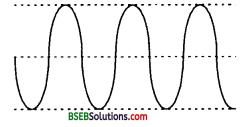Numerical Problems

Question 1.
The number of electron-hole-pairs in an intrinsic semiconductor is 2 x 1019 m-3 at 27°C and Eg is 1 eV. Calculate the number of electron hole pairs at 227°C. Give that Boltzmann constant is 8.65 x 10-5 eV/K.
Here,Taking natural log on both sides of equation (3), we get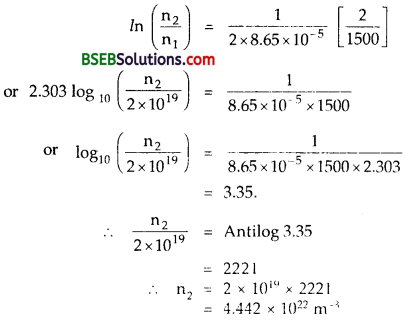Question 2.
If the above semi conductor in Q. 1. is doped by a donor impurity such that the number of conduction electrons become 2 x 1024 m-3, calculate the number of holes at 27°C. Also approximately calculate the dopant concentration.
Here,
n. = 2 x 1024 m-3
ni = 2 x 1019 m-3
nh = ?
Since ne nh = n2h
∴ nh = $$\frac{n_{1}^{2}}{n_{e}}$$
= $$\frac{\left(2 \times 10^{19}\right)^{2}}{2 \times 10^{24}}$$
= 2 x 1014m-3.
As the dopant concentration,
ND ≈ ne
ND ≈ 2 x 1024 m-3.Question 3.
A battery of e.m.f. 2 V is used in the figure given here. The length of the block is 0.1 m and the area is 10-4 m-2. If the block is of intrinsic silicon at 300 K, find the electron and hole currents. What will be the magnitude of the total current? What will be the magnitude of the total current if germanium is used instead of Si?Given : for Si,
µe = 0.135 m2 υ-1 s-1
µh = 0.048 m2 υ-1 s-1
n1 = 1.5 x 1016 m-3.
for Ge, µe = 0.39 m2 υ-1 s-1
µh = 0.19 m2 υ-1 s-1
ni = 2.4 x 1019 m-3.
Here, l = 0.1m
A = 10-4m2.
V = 2V
T = 300K
For Si, µe = 0.135 m2 V-1 s-1
ni = ne = nh = 1.5 x 1016 m-3.
µh = 0.048 m2 V-1 s-1
E = $$\frac {V}{l}$$ = $$\frac {2}{0.1}$$ = 20 V m-1
υe = µeE = 0.135 x 20 = 27 ms-1Now if Ie and Ih be the currenl dueo electron and hole respectively
then
Ie = eAnee
= 1.6x 10-19x 10-4 x 1.5 x 1016 x 2.7
= 6.48 x 107 A.
and Ih = nh eA υh.
1.5 x 1016 x 1.6 x 10-19 x 10-4 x 0.96
= 2.304 x 10-7 A

∴ total current is given by,
I = Ie + Ih
= 6.48 x 10-7 + 2.304 x 10-7
= 8.784 x 10-7 A

For Ge :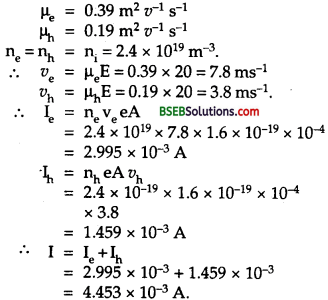Question 4.
Find the maximum wavelength of the electromagnetic radiation which can create a hole-electron pair in Ge, Eg = 0.72 eV.
Here, Eg = 0.72 eV.
= 0.72 x 1.6 x 1019 J
C = speed of light = 3 x 108ms-1
λ = maximum wavelength of the radiation which can create a hole- electron pair in Ge is given byQuestion 5.
A diode used in the circuit shown here has a constant voltage drop of 0.5 V at all currents and a maximum power rating of 100 mW. What should be the value of the resistance R connected in series with diode for obtaining maximum current?
Here, e.m.f. of the source, E = 1.5 V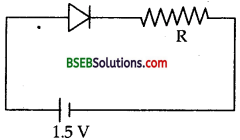Voltage drop across the diode, Vd = 0.5 V
Maximum power rating of the diode,
P = 100mW = 102 x 10-3W
= 10-1 W
= 0.1 w.
∴ Maximum current through the diode is given by
Imax = $$\frac{\mathrm{P}}{\mathrm{V}_{\mathrm{d}}}=\frac{0.1}{0.5}$$ = 0.2 A.
If V be the potential drop across R, then
V = E – Vd = 1.5 – 0.5 = 1V.
R = $$\frac{\mathrm{V}}{\mathrm{I}_{\max .}}=\frac{1}{0.2}$$ = 5 Ω

Question 6.
The base current is changed by 20 pA in a transistor. This results in a change of 0.02 V in base to emitter voltage and a change of 2 m A in the collector current.
(a) Find the input resistance, pac and the transconductance of the transistor.
(b) This transistor is used as an amplifier with the load resistance of 5 KO. What is the voltage gain of the amplifier?
Here,
∆Ib = Change in base current
= 20µA = 20 x 10-6 A.
∆Vbe = 0.02 V
∆Ic = 2mA = 2 x 10-3A.
Rin = input resistance = ?
Rin = $$\frac{\Delta \mathrm{V}_{\mathrm{be}}}{\mathrm{I}_{\mathrm{b}}}$$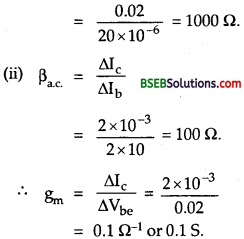(b) Here, RL = 5 KΩ = 5000 Ω
Voltage gain is given by
AV = $$\frac{\Delta \mathrm{V}_{\mathrm{ce}}}{\Delta \mathrm{V}_{\mathrm{be}}}$$
= $$\frac{\mathrm{R}_{\mathrm{L}} \times \Delta 1_{\mathrm{C}}}{\Delta \mathrm{V}_{\mathrm{be}}}$$
= $$\frac{5000 \times 2 \times 10^{-3}}{0.02}$$
= 500

Question 7.
A power supply A delivers 10 V D.C. with a ripple factor of 0.5 V r.m.s., while the power supplying delivers 25 V D.C with a ripple of 1 mV, tell which one is a better choice?
Here, for power supply A,
VAC
ripple factor = $$\frac{\mathrm{V}_{\mathrm{A} . \mathrm{C}}}{\mathrm{V}_{\mathrm{D} . \mathrm{C}}}$$
= $$\frac{0.5}{10}$$ x 100%
= 5 %
For supply B, the ripple factor is given by
= $$\frac{0.001}{25}$$
= 0.004%
Thus clearly, the power supply B is better.Question 8.
A given transistor has α = 0.98. The transistor is used in the CE configuration. If the base current is changed by 0.2 mA, then find the change in the collector current.
Here,
α = 0.98,
∴ β = $$\frac{\alpha}{1-\alpha}=\frac{0.98}{1-0.98}$$ = 49
∴ β = 49 = $$\frac{\Delta \mathrm{I}_{\mathrm{c}}}{\Delta \mathrm{I}_{\mathrm{b}}}$$
or ∆Ic = ?
∴ ∆Ic = ∆Ib x β
= 0.2 x 49 = 9.8 m.

Question 9.
If the a of a transistor changes from 0.98 to 0.99%, then calculate the percentage change in p.
When a changes from 0.98 to 0.99, then the percentage change in a is given by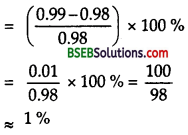Now I is given by
β = $$\frac{α}{1 – α}$$
∴Percentage change in β is
β = $$\frac{β – 1}{β}$$ x 100 %Question 10.
In a p-n-p transistor circuit, the collector current is 10 mA. If 90 % of the electrons emitted reach the collector, then calculate the emitter current and the base current.
Here,
Ic = 90 % of Ie
Ic = 90 % Ie
= $$\frac{9}{100}$$Ie
or Ic = 0.9 Ie
Also Ic = 10 mA
∴ Ie = 10 ÷ 0.9 = 11.1mA
As Ie = Ib + Ic
Ib = Ie – Ic
= 11.1 – 10
= 1.1 mA

Question 11.
A p-n junction diode when forward biased has a drop of 0.5 V which is assumed to be independent of current. The current in excess of 10 mA through the diode produces a large Joule heating which damages the diode. If we want to use a 1.5 V battery to forward bias the diode, what should be the value of resistor used in series with the diode so that the maxjmum current does not exceed 5 mA?
Let R be the resistance of the resistor used
Here,
E = 1.5 V
V = 0.5V
I = 5 mA = 5 x 10-3A
Using the relation, E = V + IR, we get
R = $$\frac{E-V}{I}=\frac{1.5-0.5}{5 \times 10^{-3}}$$
= $$\frac{1.0}{5 \times 10^{-3}}=\frac{1000}{5}$$ = 200 ΩQuestion 12.
In a Si transistor, a change of 7.89 mA in the emitter current produces a change of 7.8 mA in the collector current. What change in the base current is necessary to produce an equivalent change in the collector current?
Here,
δ Ie = Change in emitter current
= 7.89 mA.
δ Ic = Change in collector current
= 7.8mA
δ Ib = ?
Using the relation,
Ie = Ib + Ic we Set
∆Ie = ∆Ib + ∆Ic.
or ∆Ib = ∆Ie – ∆Ic
= (7.89-7.80) mA
= 0.09 mA
= 0.09 x 10-3 A
= 90 x 10-6A
= 90 μA.

Question 13.
Pure Si at 300 K has equal electron (ne and hole nh) concentration of 1.5 x 1016 m-3. Doping by indium increases nh to 4.5 x 1022 m-3. Calculate ne in doped Si.
Here, for pure Si, ne = 1.5 x 1016 m-3.
nn = 1.5 x 1016 m-3
for doped silicon,
nh = 4.5 x 1022/m3
ne = ?
ne x nh = ne2, we get
ne x nh = n’e x n’h
n’e = $$\frac{n_{e} \times n_{h}}{n_{h}}$$
= $$\frac{1.5 \times 10^{16} \times 1.5 \times 10^{16}}{4.5 \times 10^{22}}$$
= 0.5 x 1010 m-3
= 5 x 109 m-3.

Question 14.
Determine the number density of donor atoms which have to be added to an intrinsic arc semiconductor to produce an n-type semi conductor of conductivity 5 Ω-1 cm-1, given that the mobility of electrons in n-type Ge is 3900 cm2/VS. Neglect the contribution of holes to conductivity.
Here, for n-type Ge,
μe = 3900 Cm2/VS = 0.39 m2/VS.
o = 5 Ω-1 C-1 = 500 Ω-1 m-1
e = 1.6 x 10-19 C
ne = ? σh = 0
Using the relation,
σ = σe + σh
= σe + 0 = σ = eneμe
ne = $$\frac{\sigma}{\mathrm{e} \mu_{\mathrm{e}}}$$
$$\frac{500}{1.6 \times 10^{-19} \times 0.39}$$
$$\frac{5000}{16 \times 39} \times 10^{21}$$
$$\frac{5000}{624} \times 10^{21}$$
= 8.0 x 1021 m-3.Question 15.
In a common base transistor amplifier, if the input resistance is 200 Ωs and load resistance is 20 KΩ. Find (i) Voltage gain (ii) Power gain; current gain of transistor = 0.95.
Here, α = 0.95
RL = load resistance = 20 KW
= 20 x 103
Ri = input resistance = 200 Ωs.

(i) A v = ?
Using the relation,
A v = $$\frac{V_{0}}{V_{i}}=\frac{I_{C} R_{L}}{I_{e} R_{j}}=\alpha \frac{R_{L}}{R_{i}}$$
= 0.95 x $$\frac{20 \times 10^{3}}{200}$$
= 95

(ii) Power gain = ?
We know that
Power gain = α2 $$\frac{\mathrm{R}_{\mathrm{L}}}{\mathrm{R}_{\mathrm{i}}}$$
= $$(0.95)^{2} \frac{20 \times 10^{3}}{200}$$
= 0.9025 x 100
= 90.25.

Question 16.
Identify the logic gates marked C and D in the figure here. Write down the output at Y, when A = 1, B = 1 and A = 0, B = 0. How this circuit behaves?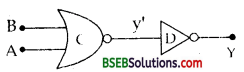Here, the logic gate marked as C is NOR gate and the logic gate marked as Y is NOT gate.
Let y’ be the output of NOR gate X. The truth table and the output y for the above mentioned inputs is given below :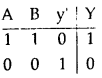This circuit behaves as ana or gate.

Question 17.
Identify the logic gates marked C, D in the figure given below and write down the output at Y when A = 1, B = 1, and A = 0, B = 1Here, the logic gate marked C is a NAND gate and D is OR gate. Let y’ be the output of NAND gate corresponding to the given inputs, y’ and y so obtained are given in the truth table.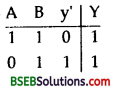Question 18.
Produce the truth table of the combination of gates shown below :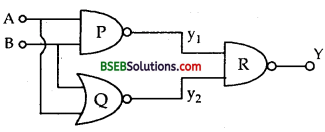Here, the gates marked P, Q, R are NAND, NOR and NAND gates respectively.
The outputs y1 and y2 are given below in the truth table 1 and the truth table of the combination is also shown in this table with output Y.i.e., the output of NAND gate is high when one or both the inputs are low and the output is low when both the inputs are high. For NOR gate, the output is high when both the inputs are low and the output is low when one or both the inputs are high.

Fill In The Blanks

Question 1.
…………. is called as junction in p-n junction diode.
The surface of contact of p-type and n-type crystal.

Question 2.
The thickness of the depletion layer is of the order of ………….
1 pm = 10-6 m.

Question 3.
The potential barrier for S, is about and the electric field across the junction is about ………….
0.7V, 7 x 105 Vm-1

Question 4.
The width of the depletion layer and the potential barrier across the junction …………. due to reverse biasing.
increases.Question 5.
The zener voltage can have value from …………. to …………. volts.
1V, several hundred.

Question 6.
The arrow in the symbol of a transistor shows the direction
Conventional current or hole current.

Question 7.
Below knee voltage, the variation of current in the p-n junction is …………. and above it, it is ………….
non-linear, linear.

Question 8.
Various components such as resistors, inductors, capacitors, transistors, diodes and logic-gates etc. are grown over one ………….
Semiconductor chipQuestion 9.
…………. is the most developing area where semiconductors are used.
Computer

Question 10.
and 0 are called ………….
bits.

Question 11.
The connection between logic and mathematics was realised by and the algebra developed by him based on 0 and 1 is called ………….
Boole, Boolean Algebra.

Question 12.
When a p-n junction is forward biased, then the motion of charge carriers across the barrier is due to …………. and when it is reversed biased, then the motion of charge carriers is due to ………….
diffusion, drift.

Question 13.
Digital circuits can be obtained by repetitive use of …………. gates and are called digital building blocks.
NAND and NOR

Question 14.
In a digital circuit, the diodes and transistors are operated by a 5V supply. The states 1 and 0 will correspond to …………. V and V …………. respectively.
5,0

Question 15.
In insulator, the valence elctrons form a band that is ………….
valence band which is filled completely.

Question 16.
In the band structure of an intrinsic semiconductor is located ………….
midway between the valence band and the conduction band.

Question 17.
The region near the junction of pn-diode where there are no charge carriers is called ………….
depletion region

Question 18.
A small impurity is added to Ge to get a p-type semiconductor and this impurity is called ………….